# Test: Transfer Function

## 10 Questions MCQ Test Topicwise Question Bank for Electronics Engineering | Test: Transfer Function

Description
Attempt Test: Transfer Function | 10 questions in 30 minutes | Mock test for Electronics and Communication Engineering (ECE) preparation | Free important questions MCQ to study Topicwise Question Bank for Electronics Engineering for Electronics and Communication Engineering (ECE) Exam | Download free PDF with solutions
*Multiple options can be correct
QUESTION: 1

### Non-minimum phase transfer function is defined as the transfer function

Solution:

The non-minimum phase transfer function is defined as the transfer function is defined as the transfer function which has zeros (or) poles in right side of s-plane

QUESTION: 2

### The type and order of the system described by the open loop transfer function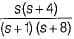are respectively

Solution: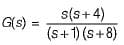There is no open loop pole at origin. Hence, type of system = 0.
Highest power of s of the characteristic equation 1+ G(s) = 0 will be 2.
Hence, order of given System = 2.

QUESTION: 3

### Assertion (A): If the number of zeros are less than the number of poles (i.e. Z < P), we say that there are zeros at infinity and the order of such zeros is P-Z Reason (R): The value of the transfer function becomes zero for s tends to zero.

Solution:

For Z < P,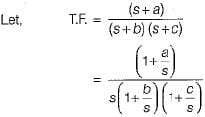∴ Here, number of zeros = 1 and no. of poles = 2
∴ P - Z = 1
When s →∞, transfer function becomes zero. Thus, there is one zero (P - Z = 1) at infinity. Thus, assertion is true.
Since value of transfer function becomes zero as s →∞ therefore, reason is false.

QUESTION: 4

The transfer function ofthe network shown below is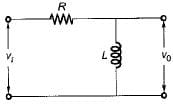Solution:

Let i be the current in the given circuit.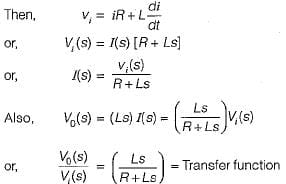QUESTION: 5

The principle of homogeneity and superposition are applied to

Solution:

Superposition theorem states that for two signals additivity and homogeneity property must be satisfied and that is applicable for the LTI systems.

QUESTION: 6

​The differential equation of a control system having input x(t) and output y(t) is given as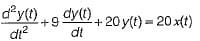The output response of the system for unit step input is given by

Solution:

Given differential equation is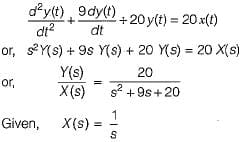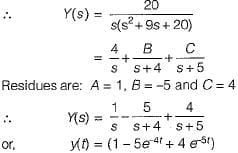QUESTION: 7

The step response of a system is given by
c(t) = 1 + 0.25 e-50t - 1.25e-10t
The steady state gain of the transfer function in time constant form will be

Solution:

Given, c(t) = 1 + 0.25 e-50t - 1.25e-10t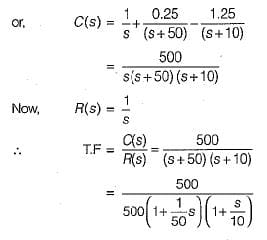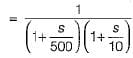Thus,C(s)/R(s) is in time constant form having steady state gain = 1.

QUESTION: 8

The poles and zeros of the transfer function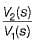for the network shown below are located at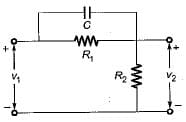Solution:

For given network, total input impedance is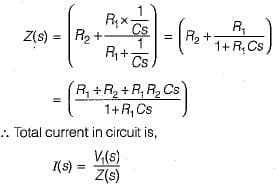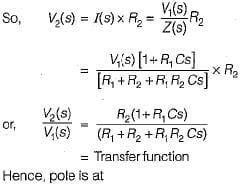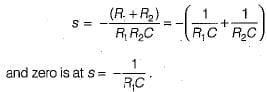QUESTION: 9

The pole-zero configuration of a transfer function is shown below: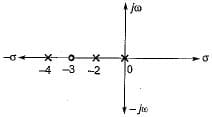If the value of the transfer function at s = 1 is 3.2, the gain factor K is

Solution:

From given pole-zero plot, transfer function is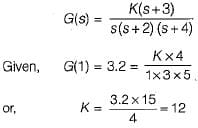So, gain factor K =12

QUESTION: 10

The type of a system denotes the number of

Solution:

The type of a system denotes the no. of poles of origin.Use Code STAYHOME200 and get INR 200 additional OFF Use Coupon Code